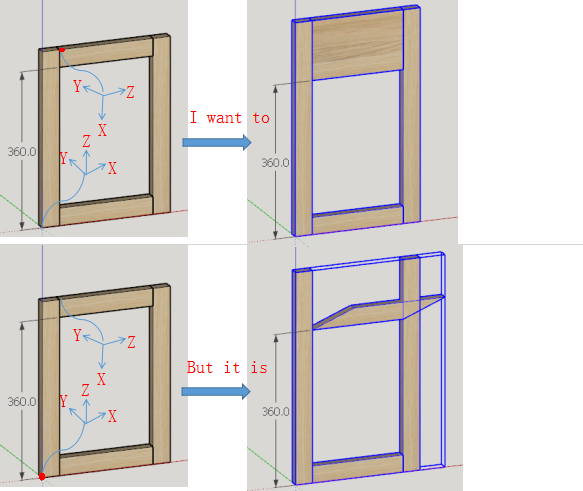# Can anyone help me with move vertices``````mod = Sketchup.active_model
ent = mod.entities
sel = mod.selection
mass = []
arr = []
sel.make_unique
sel.entities.each { |entity| arr << entity.make_unique}
for i in 0..arr.length-1
t = arr[i].transformation
arr[i].entities.to_a.each{|line|
if line.class == Sketchup::Edge
if ((line.start.position.transform! t).z > 380.mm)
mass <<  line.start
end
end
if line.class == Sketchup::Edge
if (line.end.position.transform! t).z > 380.mm
mass <<  line.end
end
end
}
pt = Geom::Transformation.new([0, 0, 100.mm])
arr[i].entities.transform_entities(pt,mass)
mass = []
end
``````

Hi, If you change the local axis of the top middle section so the Z axis points UP instead of Right, then you might achieve moving the vertices Up instead of Right.

2 Likes

… try this …

``````inst = sel.make_unique
ents = inst.definition.entities.map { |entity| entity.make_unique }
for child in ents
# code using child ...
``````

This way you avoid any “off by one errors” and since your not really using the index for any calculations you might as well just use a reference to the child instance rather than repeatedly calling `arr[i]`.

You are right, but the local axis is not fixed.

I tried and the result was the same. Is there any way to change the axes?

``````mod = Sketchup.active_model
ent = mod.entities
sel = mod.selection
mass = []
inst = sel.make_unique
ents = inst.definition.entities.map { |entity| entity.make_unique }
for child in ents
t = child.transformation
if child.transformation.zaxis != (0,0,1)
#Is there any way to change the axes?
child.axes.set([0,0,0], xaxis, yaxis, Z_AXIS) #
end

child.entities.to_a.each{|line|
if line.class == Sketchup::Edge
if (line.start.position.transform! t).z > 380.mm
mass <<  line.start
end
end
if line.class == Sketchup::Edge
if (line.end.position.transform! t).z > 380.mm
mass <<  line.end
end
end
}
pt = Geom::Transformation.new([0, 0, 100.mm])
child.entities.transform_entities(pt,mass)
mass = []
end
``````

I do not have your model, so I just guessing…

``````mod = Sketchup.active_model
ent = mod.entities
sel = mod.selection
mass = []
inst = sel.make_unique
ents = inst.definition.entities.map { |entity| entity.make_unique }
for child in ents
t = child.transformation
if t.zaxis != [0,0,1]
pt = Geom::Transformation.new([-100.mm, 0, 0])
else
pt = Geom::Transformation.new([0, 0, 100.mm])
end

child.entities.to_a.each{|line|
if line.class == Sketchup::Edge
if (line.start.position.transform! t).z > 380.mm
mass <<  line.start
end
end
if line.class == Sketchup::Edge
if (line.end.position.transform! t).z > 380.mm
mass <<  line.end
end
end
}

child.entities.transform_entities(pt, mass)
mass = []
end
``````

Yes. You need apply certain transformation the group/component in a question to align its axes, then apply the inverse of that certain transformation to the entities of group/component.

Note that `child.entities` will only be successful if the `child` is a group object.
Component Instances do not have a `#entities` convenience method.

Safer to use `child.definition.entities` for both cases.

2 Likes

nice, that worked.

How to do it, I want to know.thanks!

or:

Search results for ‘change axes #developers:ruby-api’ - SketchUp Community

1 Like